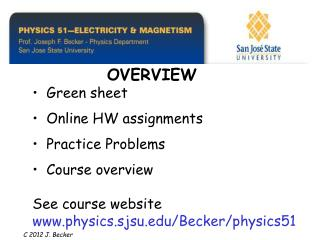# OVERVIEW - PowerPoint PPT PresentationDownload PresentationOVERVIEW

OVERVIEW
Download Presentation## OVERVIEW

- - - - - - - - - - - - - - - - - - - - - - - - - - - E N D - - - - - - - - - - - - - - - - - - - - - - - - - - -
##### Presentation Transcript

1. OVERVIEW • Green sheet • Online HW assignments • Practice Problems • Course overviewSee course website www.physics.sjsu.edu/Becker/physics51 C 2012 J. Becker

2. PHYSICS 51 GREEN SHEET Front side: Course outline Back side: Reading assignment and problems

3. 3 slides printed on a page

4. Chapter 21A Electric Field and Coulomb’s Law • Electric charge (sec. 21.1) • Conductors, insulators, and induced charge (sec. 21.2) • Coulomb’s Law (sec. 21.3) • Electric field lines (sec. 21.6) C 2012 J. Becker

5. Learning Goals - we will learn: • The nature of electric charge.• How objects become electrically charged.• How to use Coulomb’s Law to calculate the electric force between charges.• How to calculate the electric field caused by electric charges.• How to use the idea of electric field lines to visualize electric fields.

6. Electric charge • Protons have positive charge • Electrons have negative charge • Opposite signs attract • Similar signs repel • Electric field – used to calculate force between charges C 2012 J. Becker

7. Photocopiers are amazing devices. They use electric charge to hold fine dust (toner) in patterns until the pattern may be transferred to paper and made permanent with heat.

8. LITHIUM (Li) ELEMENTAtom: electrically neutral 3 protons and 3 elec. Positive ion: missing one electron so net charge is positiveNegative ion: has added electron so net charge is negative

9. A positive charge and a negative charge attract each other. Two positive charges (or two negative charges) repel each other.

10. Electric forces in action Figure 21.5

11. Figure 21.1a

12. Figure 21.1b

13. Figure 21.1c

14. Copper is a good conductor of electricity;Glass and nylon are good insulators Figure 21.6a

15. Figure 21.6b

16. Figure 21.6c

17. CHARGING A METAL SPHERE BY INDUCTION Charges are free to move in a conductor but are tightly bound in an insulator. The earth (“ground”) is a large conductor having many free charges.

18. POLARIZED insulator

19. CHARGED COMB ATTRACTS A PIECE OF PAPER In an insulator the charges can move slightly (called polarization of the insulator). A piece of paper is attracted to a charged comb because the positive charges are closer to the negatively charged comb (in the upper figure).

20. e - PAPER DISPLAY (Kindle, i - PAD, etc.)wikipedia.org/wiki/Electronic_paper Scheme of an electrophoretic display.

21. e - PAPER DISPLAY (Kindle, i - PAD, etc.)wikipedia.org/wiki/Electronic_paper Each capsule contains an oily solution containing black dye (the electronic ink), with numerous white titanium dioxide (white) particles suspended within. The white particles are slightly negatively charged. Applying a negative charge to the surface electrode repels the particles to the bottom of local capsules, forcing the black dye to the surface and giving the capsule, or pixel, a black appearance. Reversing the voltage has the opposite effect - the particles are forced to the surface, giving the pixel a white appearance.

22. An uncharged conductor can attract the charge imparted to paint droplets. Excess charges can flow to or from “ground” Car door -e

23. The imaging drum is aluminum coated with selenium, which changes from an insulator to a conductor when illuminated with light. Figure 21.2 LASER PRINTER USES CHARGED TONER

24. FORCEbetween two charges is given by Coulomb’s Law: | F | = k | Q qo| / r2

25. We can use our notion of the gravitational field to form the concept of an ELECTRIC FIELD (E) Recall force between two masses: F = m gg is the gravitational field (9.8 m/sec2) | F | = G | M m | / r2 The force between two charges Q and qo is given by: F = qoE | F | = k | Q qo | / r2

26. Coulomb’s Law:|F | = k | Q qo| / r2 Rearranged:|F | = | qo [k Q/r2]| Gives us:F = qoE where the electric field E is: | E | = | k Q / r2|

27. ELECTRIC FIELD LINES START AND END AT ELECTRIC CHARGES An electric charge is surrounded by an electric field just as a mass is surrounded by a gravitational field.

28. Electric field and equipotential lines are perpendicular to each other In Lab #2 a voltmeter is used to measure the equipotential lines (in Volts) in order to determine the magnitude and direction of the electric field lines.

29. Forces on electron beam in a TV tube (CRT) F = Q E and F = m g (vector equations)

30. Review see www.physics.sjsu.edu/Becker/physics51

31. INTRODUCTION: see Ch. 1 Vectors Review (See Chapter 1) Used extensively throughout course C 2012 J. Becker

32. Vectors are quantities that have both magnitude and direction. An example of a vector quantity is velocity. A velocity has both magnitude (speed) and direction, say 60 miles per hour in a DIRECTION due west. (A scalar quantity is different; it has only magnitude – mass, time, temperature, etc.)

33. The scalar (or dot) product of two vectors is defined as Note: The dot product of two vectors is a scalar quantity.

34. The vector (or cross) product of two vectors is a vector where the direction of the vector product is given by the right-hand rule. The MAGNITUDE of the vector product is given by:

35. Figure 21.14

36. PROFESSIONAL FORMAT